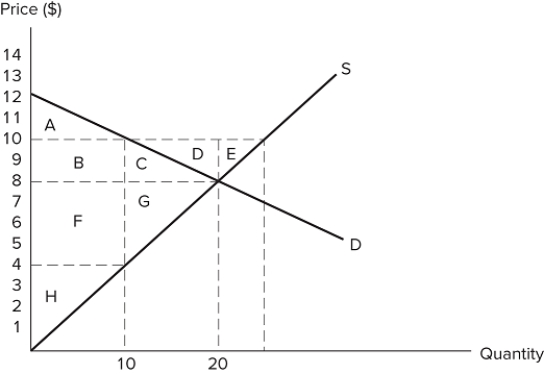Try out our new practice tests completely free!Solved

# According to the Graph Shown, If the Market Goes from Equilibrium

Question 108
Multiple Choice
Multiple Choice

##According to the graph shown, if the market goes from equilibrium to having its price set at \$10 producer surplus will change:

A) from (F + G + H) to (B + C + D + E + F + G + H).
B) from (F + G + H) to (B + C + F + G + H).
C) from (F + G + H) to (B + F + H).
D) from (C + G) to (B + C + F + G + H).

Choose question tag10+ million students use Quizplus to study and prepare for their homework, quizzes and exams through 20m+ questions in 300k quizzes.

Explore our library and get Economics Homework Help with various study sets and a huge amount of quizzes and questions

Get Free Access Now!Textbook Solutions

Find all the solutions to your textbooks, reveal answers you would’t find elsewhereSearch By Image

Scan any paper and upload it to find exam solutions and many moreFlashcards

Studying is made a lot easier and more fun with our online flashcards